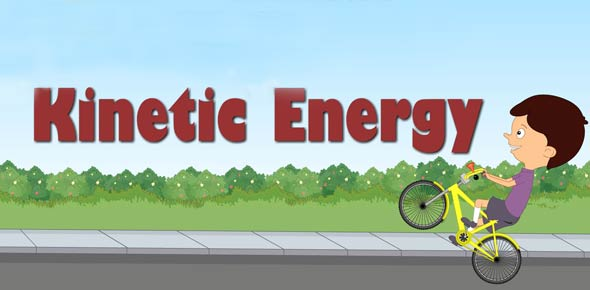# Kinetic And Potential Energy

10 Questions | Total Attempts: 1357SettingsKinetic Energy and Potential Energy

• 1.
Decide whether this has kinetic or potential
• A.

KINETIC

• B.

POTENTIAL

• 2.
• A.

KINETIC

• B.

POTENTIAL

• 3.
• A.

KINETIC

• B.

POTENTIAL

• 4.
• A.

KINETIC

• B.

POTENTAIL

• 5.
• A.

KINETIC

• B.

POTENTIAL

• 6.
• A.

KINETIC

• B.

POTENTIAL

• 7.
• A.

KINETIC

• B.

POTENTIAL

• 8.
• A.

KINETIC

• B.

POTENTIAL

• 9.
Kinetic Energy is energy which is moving and therefore being used.
• A.

True

• B.

False

• 10.
Potential energy is the energy of an object because of it's position (resting or waiting). It is also called possible energy.
• A.

True

• B.

False

Related TopicsBack to top